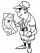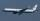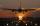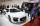The cruise ship

The cruise ship has a speed of 12 km / h at a calm surface. When we sail 45 km along the river and 45 km back, it took us exactly 8 hours. Which (constant) speed of flow of the river?

Result

v =  3 km/h

Solution:Checkout calculation with our calculator of quadratic equations.

Leave us a comment of this math problem and its solution (i.e. if it is still somewhat unclear...):Be the first to comment!To solve this verbal math problem are needed these knowledge from mathematics:

Looking for help with calculating roots of a quadratic equation? Do you have a linear equation or system of equations and looking for its solution? Or do you have quadratic equation?

Next similar math problems:

1. Tourist JirkaDistance between the points A and B is 13.5 km. Jirka went from point A to point B unknown speed and for an unknown period of time. Back to the point A went slower by 3 km/h which means that went 20 minutes more. How long Jirka took the return journey?
2. Two planesTwo planes flying from airports A and B, 420 km distant against each other. Plane from A took off 15 minutes later and flies at an average speed of 40 km/h higher than the plane from B. Determine the average speed of this two aircraft if you know that it
3. Wind driftThe plane flies at 860 km/h, passing distance 3000 kilometers with the wind and once again against the wind for 6 h 59 min. What is the wind speed?
4. Speed of carThe car went to a city that was 240 km away. If his speed increased by 8 km/h, it would reach the finish one hour earlier. Determine its original speed.
5. Theorem proveWe want to prove the sentence: If the natural number n is divisible by six, then n is divisible by three. From what assumption we started?
6. Solve 3Solve quadratic equation: (6n+1) (4n-1) = 3n2
7. Square root 2If the square root of 3m2 +22 and -x = 0, and x=7, what is m?
8. Evaluation of expressionsIf a2-3a+1=0, find (i)a2+1/a2 (ii) a3+1/a3
9. DiscriminantDetermine the discriminant of the equation: ?
10. RootsDetermine the quadratic equation absolute coefficient q, that the equation has a real double root and the root x calculate: ?
11. EquationEquation ? has one root x1 = 8. Determine the coefficient b and the second root x2.Find the roots of the quadratic equation: 3x2-4x + (-4) = 0.solve equation: ?Find variable P: PP plus P x P plus P = 160In cinema are 1656 seats and in the last row are 105 seats , in each next row 3 seats less. How many are the total rows in cinema?Quadratic equation ? has roots x1 = -26 and x2 = -86. Calculate the coefficients b and c.Solve this equation: x + 5/x - 6 = 4/11